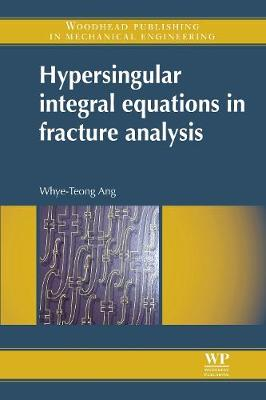•Hypersingular Integral Equations in Fracture Analysis (Hardback)

(author)
£138.00
Hardback 212 Pages / Published: 10/12/2013
• We can order this

Usually dispatched within 3 weeks

Hypersingular Integral Equations in Fracture Analysis explains how plane elastostatic crack problems may be formulated and solved in terms of hypersingular integral equations. The unknown functions in the hypersingular integral equations are the crack opening displacements. Once the hypersingular integral equations are solved, the crack tip stress intensity factors, which play an important role in fracture analysis, may be easily computed. This title consists of six chapters: Elastic crack problems, fracture mechanics, equations of elasticity and finite-part integrals; Hypersingular integral equations for coplanar cracks in anisotropic elastic media; Numerical methods for solving hypersingular integral equations; Hypersingular boundary integral equation method for planar cracks in an anisotropic elastic body; A numerical Green's function boundary integral approach for crack problems; and Edge and curved cracks and piezoelectric cracks. This book provides a clear account of the hypersingular integral approach for fracture analysis, gives in complete form the hypersingular integral equations for selected crack problems, and lists FORTRAN programs of numerical methods for solving hypersingular integral equations.

Publisher: Elsevier Science & Technology
ISBN: 9780857094797
Number of pages: 212
Weight: 480 g
Dimensions: 234 x 156 x 13 mm